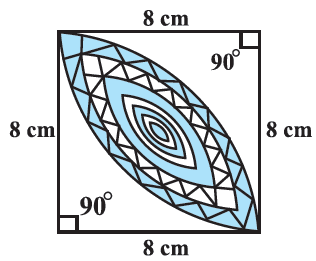In the verge of coronavirus pandemic, we are providing FREE access to our entire Online Curriculum to ensure Learning Doesn't STOP!

# Ex.12.3 Q16 Areas Related to Circles Solution - NCERT Maths Class 10

Go back to  'Ex.12.3'

## Question

Calculate the area of the designed region in Figure common between the two quadrants of circles of radius $$\text{8 cm}$$ each.Video Solution
Areas Related To Circles
Ex 12.3 | Question 16

## Text Solution

What is  known?

Designed region is the common area between the $$2$$ quadrants of circles of radius $$\text{8 cm.}$$

What is unknown?

Area of the designed region.

Reasoning:

In a circle with radius $$r$$ and angle at the center with degree measure $$\theta$$;

(i) Area of the sector \begin{align} = \frac{{\rm{\theta }}}{{{{360}^{\rm{\circ}}}}} \times \pi {r^2}\end{align}

(ii) Area of the segment = Area of the sector - Area of the corresponding triangle

From the figure,it is observed that

Area of the designed region = $$2\times$$Area of the segment of the quadrant of radius $$\rm8\,cm$$

$$2\times$$[Area of the quadrant - Area of the right triangle]

\begin{align} = \frac{{{{90}^ \circ }}}{{{{360}^ \circ }}} \times \pi {r^2} = \frac{1}{4}\pi {r^2}\end{align}

Area of the right triangle

\begin{align} = \frac{1}{2} \times \text{base} \times \text{height} \end{align}

Steps:

From the figure, it is observed that

Area of the designed region $$=2\times$$Area of the segment of the quadrant of radius $$\rm{}8\,cm$$

$$=2\times$$[Area of the quadrant - Area of the right triangle]

\begin{align}&= \!2\! \times\! \left[ \frac{1}{4}\pi {r^2} \!- \!\frac{1}{2} \!\times\! \text{base}\! \times\! \text{height} \right] \\&= \!2 \!\times\! \left[ \frac{1}{4} \!\times\! \frac{{22}}{7} \!\times\! 8 \rm cm \!\times\! 8cm \! - \!\frac{1}{2} \!\times\! \rm 8cm \!\times\! 8 \rm cm \right] \\&= \!2 \!\times\! \left[ {\frac{{352}}{7} \rm c{m^2} \!- \!32c{m^2}} \right]\\&= \!2 \!\times\! \left[ {\frac{{352 \!-\! 224}}{7} \rm c{m^2}} \right]\\&=\! 2 \times \frac{{128}}{7} \rm c{m^2}\\&= \frac{{256}}{7} \rm c{m^2}\end{align}

Learn from the best math teachers and top your exams

• Live one on one classroom and doubt clearing
• Practice worksheets in and after class for conceptual clarity
• Personalized curriculum to keep up with school